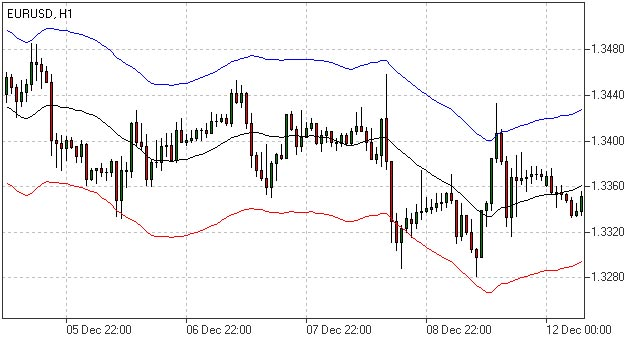Envelopes Indicator - Moving Average Envelope

Envelopes Indicator Definition

The Envelopes indicator reflects the price overbought and oversold conditions helping to identify the entry or exit points as well as possible trend break-downs.

Test the Indicator in Action
Once opened Demo you will be supplied with educational materials and online support in your own language

How to Use Envelopes Indicator

The Envelopes indicator consists of two SMAs that together form a flexible channel in which the price evolves. The averages are plotted around a Moving Average in a constant percentage distance which may be adjusted according to the current market volatility. Each line serves as a margin of the price fluctuation range.

In a trending market take only oversold signals in an uptrend conditions and overbought signals in a downtrend conditions.

In a ranging market the price reaching the top line serves as a sell signal, while the price at the lower line generates a signal to buy.Envelopes Indicator

Envelopes Indicator Formula (Calculation)

In the calculation of the upper and lower lines of the Envelopes indicator, the volume of deviation from the moving average is set according to the average volatility of the instrument (the higher it is, the great the deviation).

Here is the formula for calculating the Envelopes Indicator:

Upper Band = SMA(CLOSE, N)*[1+K/1000]
Lower Band = SMA(CLOSE, N)*[1-K/1000]

In the Envelopes Indicator formula, the SMA is the Simple Moving Average, the N is the averaging period, the K/1000 is the value of shifting from the average (measured in basis points).